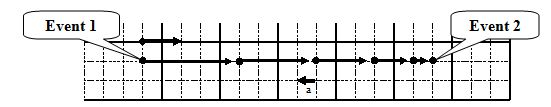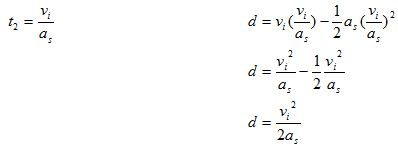$$\require{cancel}$$

# 06. Symbolic Analysis

•• Contributed by Paul D'Alessandris
• Professor (Engineering Science and Physics) at Monroe Community College

# No numbers – just variables

Working the problem out using only variables will save you time and a sore hand. The object here is to first recognize what you need to solve for. Then solve for the variable without numbers, so that you will have your equation in the form that best models what you need to use it for. Causeing you to just plug in all of your numbers and pop out an answer, without having to move long decimals and complex fractions to each side of the equation. Therefore solving your problem with minimal error and using less time.

Consider the following situation:

The driver of an automobile suddenly sees an obstacle blocking her lane. Determine the total distance the auto travels between seeing the obstacle and stopping (d) as a function of the initial velocity of the car (vi) and the magnitude of its acceleration while stopping (as).

As always, the first step in analyzing motion is to draw a motion diagram.Rather than calculate the stopping distance for particular values of initial velocity and acceleration, the goal of this activity is to determine, in general, how the stopping distance depends on these two parameters. If we can construct this function, we can then use the result to calculate the stopping distance for any car if we know its initial velocity and stopping acceleration.

Although this sounds like a different task from what we’ve done in the previous two examples, we will approach this task exactly the same way, by tabulating what we know about the situation,

 Event 1: The instant the driver hits the brakes. t1 = 0 s r1 = 0 m v1 = vi a12 = -as Event 2: The instant the car stops. t2 = r2 = d v2 = 0 m/s

and then applying our two kinematic relationships:Since our goal is to determine d as a function of vi and as, we must eliminate t2. To do this, solve for t2 in the left equation and substitute this expression into the right equation.Thus, the stopping distance appears to be proportional to the square of the initial velocity and inversely proportional to the stopping acceleration. Does this make sense?

To determine if a symbolic expression is sensible, it is often useful to check limiting cases. A limiting case is when one of the variables in the expression takes on an extreme value, typically zero or infinity. For example, if the initial velocity of the car was zero the stopping distance would have to also be zero, since the car was never moving! Allowing vi to equal zero in the above expression results in a stopping distance of zero, so our expression “passes” this logical test. Another limiting case would be setting the acceleration of the car equal to zero. With no acceleration, the car should never stop. In our expression, setting the acceleration equal to zero results in an infinite stopping distance, which again agrees with commonsense. If our expression did nt give the correct results in these limiting cases, we would know we made an error somewhere in the derivation (and, of course, we would then go back and find our mistake and fix it because we are good students …).